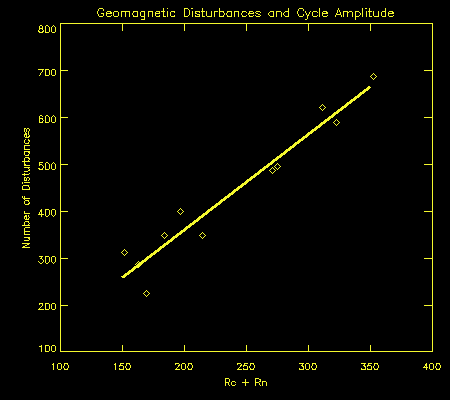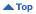Educational
HomeEducationalThe Sun and Solar ActivitySolar CycleThe Amplitude of a Solar Cycle Thursday, Dec 02 2021 03:45 UT

# Section Information

The Sun and Solar Activity

# The Amplitude of a Solar Cycle

Many methods are used to predict the amplitude of a solar cycle prior to its onset but only the precursor technique has won approval from the scientific community. This technique works on the notion that the solar cycle begins some years before solar minimum with the appearance of long-lived, coronal holes which give rise to sequences of 27-day recurrent geomagnetic disturbances.

Many applications of the precursor method use the long record of geomagnetic disturbances to correlate their occurrence with the amplitude of the next solar cycle. But there are two limitations. Firstly, even in the declining phase geomagnetic disturbances can result from other sources making it necessary to correct for disturbances arising from current cycle events such as coronal mass ejections. Secondly, the time of onset of recurrent geomagnetic activity seems to vary from cycle to cycle. Any method which relies on activity over (say) the last three years of the cycle may incorrectly estimate the precursor activity and the amplitude of the new cycle.

Thompson (Solar Physics, Vol. 148, page 383, 1993) has developed a version of the precursor method to avoid both of these limitations. The technique is simple: it correlates the total number of geomagnetic disturbances in each cycle (solar minimum to the next solar minimum) with two parameters - the amplitude of the current cycle and that of the next. When a geomagnetic disturbance is defined as a day with Ap >= 25 (or statistically equivalent value for the aa index for earlier data) it is found that both cycles are close to equally important in determining the number of disturbed days. The equation obtained is:

Nc = -47.9 + 2.20 Rc + 1.88 Rn

where, Nc is the number of geomagnetic disturbances in the current cycle, Rc is the amplitude in sunspot number of the current cycle and Rn is the amplitude of the next cycle.

The figure below shows the number of disturbed days (Ap >= 25) plotted against (for display purposes) the sum of the amplitudes of Cycle 22 and the Cycle 23. The good relationship shows that the number of disturbed days in a solar cycle is very well determined by the two amplitudes.

This good relationship gives a method of predicting the amplitude of the next cycle because at solar minimum we know the number of disturbed days and the amplitude of the cycle just ended. Thompson has applied this method to predict the amplitude of 11 previous cycles producing a r.m.s. error in the amplitude of only 17 in sunspot number.

What are the prospects for Cycle 23 using this method? A final prediction can only be made at solar minimum but, knowing the number of disturbed days so far in the cycle, a lower limit estimate can be made. This estimate builds to a final prediction at solar minimum.

Using Ap data up to February 1996 we find for the amplitude of Cycle 23

R23 >= 161

With solar minimum likely in the next few months this lower limit will increase only slightly. Hence, the prediction suggests that Cycle 23 will be a very large solar cycle comparable to Cycle 21 in amplitude but short of the record Cycle 19.Material prepared by Richard Thompson